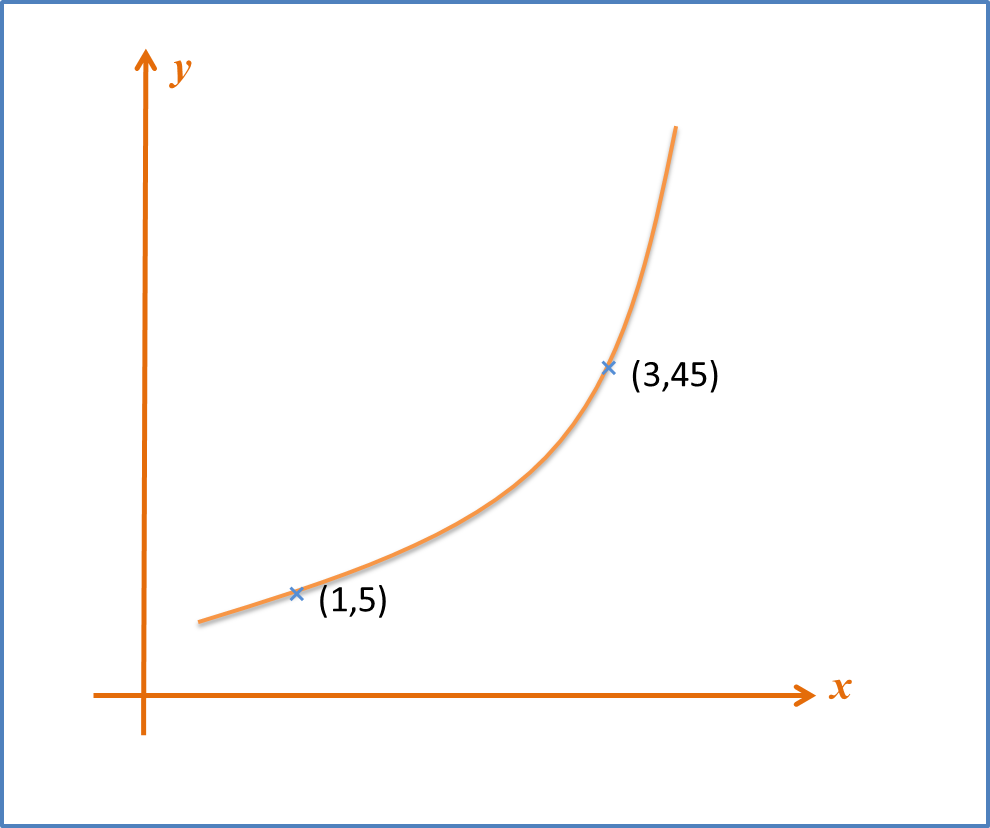\

# SPM Practice 2 (Question 6 & 7)

Question 6:
The variables x and y are related by the equation $y=p{x}^{3}$, where p is a constant. Find the value of p and n.Solution:Question 7:
Diagram A shows part of the curve $y=a{x}^{2}+bx$ .  Diagram B shows part of the straight line obtained when the equation is reduced to the linear form. Find
(a) the values of a and b,
(b) the values of p and q.Diagram ADiagram B

Solution: